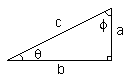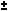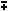We are here with you hands in hands to facilitate your learning & don't appreciate the idea of copying or replicating solutions. Read More>>

#www.vustudents.ning.com

Looking For Something at vustudents.ning.com? Click Here to Search

 www.bit.ly/vucodes + Link For Assignments, GDBs & Online Quizzes Solution www.bit.ly/papersvu + Link For Past Papers, Solved MCQs, Short Notes & More

DON’T MISS THESE: Important instructions before attempting the solution of this assignment:

•     To solve this assignment, you should have good command over 09 - 13 lectures.

• Try to get the concepts, consolidate your concepts and ideas from these questions which you learn in the 09 to 13 lectures.

•     Upload assignments properly through LMS, No Assignment will be accepted through email.

•     Write your ID on the top of your solution file.

• Don’t use colorful back grounds in your solution files.
• Use Math Type or Equation Editor etc for mathematical symbols.
• You should remember that if we found the solution files of some students are same then we will reward zero marks to all those students.
• Try to make solution by yourself and protect your work from other students, otherwise you and the student who send same solution file as you will be given zero marks.
• Also remember that you are supposed to submit your assignment in Word format any other like scan images etc will not be accepted and we will give zero marks correspond to these assignments.+ Click Here To Join also Our facebook study Group...How to Join Subject Study Groups & Get Helping Material?..See Your Saved Posts Timeline

Views: 4573

.

+ http://bit.ly/vucodes (Link for Assignments, GDBs & Online Quizzes Solution)

+ http://bit.ly/papersvu (Link for Past Papers, Solved MCQs, Short Notes & More)

+ Click Here to Search (Looking For something at vustudents.ning.com?)

Attachments:

### Replies to This Discussion

Our main purpose here discussion not just Solution

We are here with you hands in hands to facilitate your learning and do not appreciate the idea of copying or replicating solutions.

 Trigonometric Identities (Math | Trig | Identities)sin(theta) = a / c csc(theta) = 1 / sin(theta) = c / a cos(theta) = b / c sec(theta) = 1 / cos(theta) = c / b tan(theta) = sin(theta) / cos(theta) = a / b cot(theta) = 1/ tan(theta) = b / a

sin(-x) = -sin(x)
csc(-x) = -csc(x)
cos(-x) = cos(x)
sec(-x) = sec(x)
tan(-x) = -tan(x)
cot(-x) = -cot(x)

 sin^2(x) + cos^2(x) = 1 tan^2(x) + 1 = sec^2(x) cot^2(x) + 1 = csc^2(x) sin(xy) = sin x cos ycos x sin y cos(xy) = cos x cosysin x sin y

tan(xy) = (tan xtan y) / (1tan x tan y)

sin(2x) = 2 sin x cos x

cos(2x) = cos^2(x) - sin^2(x) = 2 cos^2(x) - 1 = 1 - 2 sin^2(x)

tan(2x) = 2 tan(x) / (1 - tan^2(x))

sin^2(x) = 1/2 - 1/2 cos(2x)

cos^2(x) = 1/2 + 1/2 cos(2x)

sin x - sin y = 2 sin( (x - y)/2 ) cos( (x + y)/2 )

cos x - cos y = -2 sin( (x - y)/2 ) sin( (x + y)/2 )

Trig Table of Common Angles
angle 0 30 45 60 90
sin^2(a) 0/4 1/4 2/4 3/4 4/4
cos^2(a) 4/4 3/4 2/4 1/4 0/4
tan^2(a) 0/4 1/3 2/2 3/1 4/0

Given Triangle abc, with angles A,B,C; a is opposite to A, b opposite B, c opposite C:

a/sin(A) = b/sin(B) = c/sin(C) (Law of Sines)

 c^2 = a^2 + b^2 - 2ab cos(C)b^2 = a^2 + c^2 - 2ac cos(B) a^2 = b^2 + c^2 - 2bc cos(A)
(Law of Cosines)

(a - b)/(a + b) = tan [(A-B)/2] / tan [(A+B)/2] (Law of Tangents)

cos(2x) = cos^2(x) - sin^2(x) = 2 cos^2(x) - 1 = 1 - 2 sin^2(x)In other words we can “factor” a multiplicative constant out of a limit.

sec part of question  kese solve kia apne ?

Question No.3the function is continuous because limit and function are same 0 value. limit approach to 0 (so no jump)

so, it is continuous

thanx, and plz upload answer of 4th question